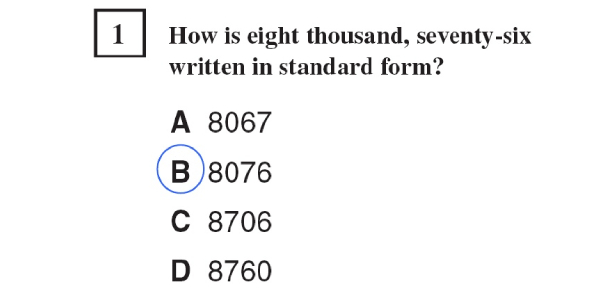# CST Math Released Questions Test

7 Questions | Total Attempts: 831SettingsMath Test

• 1.
What is 40% of 250?
• A.

A. 50

• B.

B. 100

• C.

C. 150

• D.

D. 200

• 2.
53 =
• A.

A. 5 x 5 x 5

• B.

B. 5 + 5 +5

• C.

C. 3 x 3 x 3 x 3 x 3

• D.

D. 3 + 3 + 3 + 3 +3

• 3.
• A.

A.

• B.

B.

• C.

C.

• D.

D.

• 4.
• A.

A. P

• B.

B. Q

• C.

C. R

• D.

D. S

• 5.
-7 + ( -2) = 5
• A.

True

• B.

False

• 6.
11.3 x 2.7 =
• A.

A. 29.31

• B.

B. 29.51

• C.

C. 30.31

• D.

D. 30.51

• 7.
• A.

A

• B.

B

• C.

C

• D.

D

Related TopicsBack to top